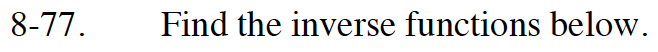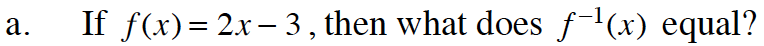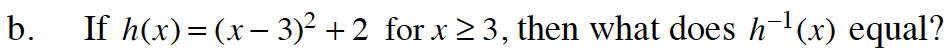Home > CCA2 > Chapter 8 > Lesson 8.2.1 > Problem8-77

8-77.
1. Find the inverse functions below. Homework Help ✎

1. If f(x) = 2x − 3, then what does f 1(x) equal?

2. If h(x) = (x − 3)2 + 2 for x ≥ 3, then what does h1(x) equal?Rewrite the equation as y = 2x − 3. Then switch x and y and solve for y.

x = 2y − 3

Solve for y.

$f^{-1}(x) = \frac{x + 3}{2}$See part (a).

$h^{-1}(x) = \sqrt{x - 2} + 3$